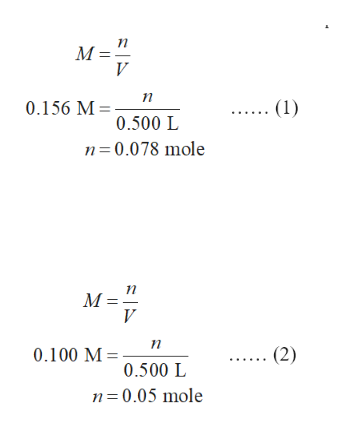# A solution is made by mixing exactly 500 mL of 0.156 M NaOH with exactly 500 mL of 0.100 MCH3COOH. Calculate the equilibrium concentration of the species below.Ka of CH3COOH is 1.8×10−5 [ H+]  ×10 MEnter your answer in scientific notation.  [ OH−]  M  [ CH3COOH]  ×10 MEnter your answer in scientific notation.  [ Na+]  M  [ CH3COO−]  M

Question
79 views

A solution is made by mixing exactly 500 mL of 0.156 M NaOH with exactly 500 mL of 0.100 MCH3COOH. Calculate the equilibrium concentration of the species below.

Ka of CH3COOH is 1.8
×
10−5

[

H+
]

×

10

M

[

OH
]

M

[

CH3COOH
]

×

10

M

[

Na+
]

M

[

CH3COO
]

M

check_circle

Step 1

The relation between milliliters (mL) and liters (L) is written below.

1 mL = 0.001 L

Convert  500 mL into liters by using the above relation.

500 mL = 0.500 L

The number of moles of NaOH is calculated in equation (1).

…… (1)

The number of moles of CH3COOH  is calculated in equation (2).help_outlineImage TranscriptioncloseM (1) 0.156 M = 0.500 L n= 0.078 mole M = п (2) 0.100 M 0.500 L n=0.05 mole fullscreen
Step 2

The number of moles of NaOH left in the solution is calculated below.

n = 0.078 mol – 0.05 mol

= 0.028 mol

The total volume of the solution is 1000 mL or 1 L. In 1 L solution, the concentration of species becomes equal to the number of moles. The total concentration of sodium ions in the solution is from sodium hydroxide(0.028 M) and sodium acetate (0.05 M). Thus,  the concentration of sodium ion is 0.078 M.

NaOH is a strong electrolyte as it completely ionizes itself. The left over concentration of  hydroxide ion is  0.028 M.

Step 3

The hydrogen concentration ...

### Want to see the full answer?

See Solution

#### Want to see this answer and more?

Solutions are written by subject experts who are available 24/7. Questions are typically answered within 1 hour.*

See Solution
*Response times may vary by subject and question.
Tagged in

### Chemistry